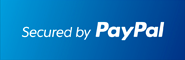** New: ECONOMICS , DIFFERENTIAL EQUATIONS **         ** SPECIALS for bulk apps **         ** Bestsellers: CALCULUS , PHYSICS , CHEM , STOCKS & PORTFOLIOS , ALGEBRA **STEP BY STEP Implicit Differentiation with examples- Learn how to do it in either 4 Steps or in just 1 Step.

A) You know how to find the derivatives of explicitly defined functions such as y=x^2 , y=sin(x) , y=1/x, etc .

What if you are asked to find the derivative of x*y=1 ? This is an Implicitly defined function (typically a relation) as y is not alone on the left side of the equation.

Well, one way would be to rewrite it as an explicit function by dividing both sides by x to get y=1/x and we know its derivative dy/dx = -1/x^2 using quotient rule.

Well, this Houdini "trick" does not always work. You couldn't use it for x^2*y+y^3*x=6. So how can we find its derivative?

First, we have to recall and use the fact that y as the dependent variable depends on x, so we could rewrite the equation as x^2*y(x)+(y(x))^3*x=6 .

This equation format convinces us i.e. to use chain rule for (y(x))^3 yielding 3*(y(x))^2*(dy/dx) where dy/dx=y'(x) is the derivative of y with respect to x.

Altogether, when applying product rule (f*g)' = f'*g + f*g' we get : 2x*y(x)+x^2*(dy/dx) + 3*(y(x))^2*(dy/dx)*x+(y(x))^3=0 (This is STEP 1)

Since we are after dy/dx , we have to factor it : (dy/dx)*[x^2 + 3*(y(x))^2] + 2x*y(x) + (y(x))^3=0 (This is STEP 2)

And we have to subtract the non dy/dx to other side: (dy/dx)*[x^2 + 3*(y(x))^2] = - 2x*y(x) - (y(x))^3 (This is STEP 3)

Lastly, we just have to divide by the non dy/dx on the left side: dy/dx = (- 2x*y(x) - (y(x))^3 ) / [x^2 + 3*(y(x))^2] (This is STEP 4)

Congrats, you just mastered Implicit Differentiation Step by Step (in 4 Steps to be precise) !!!!!

Lets revert back to y instead of y(x) : dy/dx = (-2x*y-y^3)/(x^2 + 3*y^2) to make our final derivative look less complicated. VOILA!!!

Remember that this derivative evaluated at (x,y)-points gives the slope at those points and we can draw conclusion about in/decreasing, extrema etc.

B) Lets go back to x*y=1 . Try to find its derivative using the 4 Implicit Differentiation Steps outlined above and compare it to the derivative found earlier.

Continue reading after performing the STEP BY STEP Implicit Differentiation!

STEP 1 : 1*y + x*dy/dx = 0 by Product Rule.

STEP 2 + 3: x*dy/dx = -y no factoring here and subtract the non dy/dx.

STEP 4 : dy/dx = -y/x DONE. Now how does -y/x match -1/x^2 ? Algebra helps here: Since y=1/x : -y/x = -(1/x)/x = -1/x^2 and everything that starts well ends well ;-)

C) IMPLICIT DIFFERENTATION in only 1 STEPS ? Is this Possible???? Yes it is ....

To accomplish this, we have to subtract whatever is on the right side over the left side. In our example above, we get x^2*y+y^3*x-6=0, lets name this F=0 . Easy enough.

Now we use: dy/dx = -Fx/Fy that is our little formula.

Fx = is the derivative of the left side where x is our variable and the y's are treated as constants (Think of y as y=10) . In our example, Fx= 2*x*y+y^3

Similarly, Fy = is the derivative of the left side where y is our variable and the x's are treated as constants. (Think of x as x=10) . Fy = x^2 + 3y^2*x

After this prep work , we find dy/dx = -Fx/Fy = -(2*x*y+y^3)/(x^2 + 3*y^2*x)

Now, how cool is this ?? Implicit Differentiation in only 1 STEP plus a bit of prep work.

Can you do Implicit Differentiation in 1 step for x*y=1 ? Or x*y -1 = 0

Here is how: Fx = y , Fy = x . Thus, dy/dx = -Fx/Fy = -y/x Done.

D) SUMMARY: Make an implicitly defined function explicit, if possible, by solving for y and differentiate it as such.

If impossible, do Implicit Differentiation in 4 Steps as outlined in B) .

And if you are not afraid of finding the partial derivatives Fx and Fy then do Implicit Differentiation in just 1 step. Though, your teacher may not like it as the typical 4 steps in B) are not included and your final solution looks if copied.

And here comes your Implicit Differentiation Calculator showing Step by Step solutions